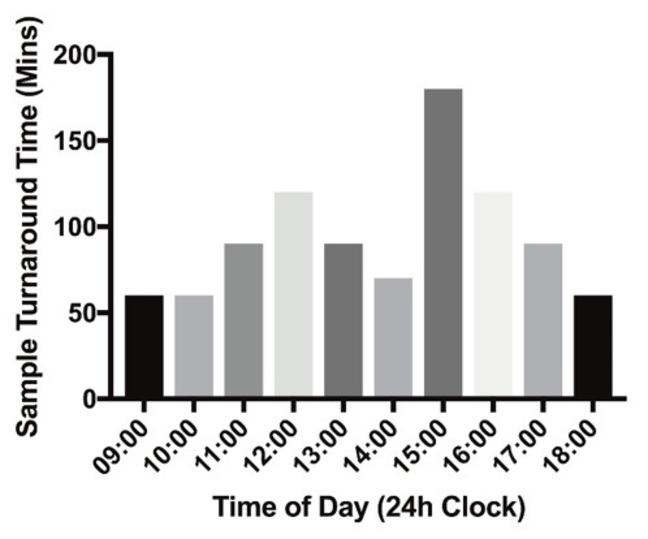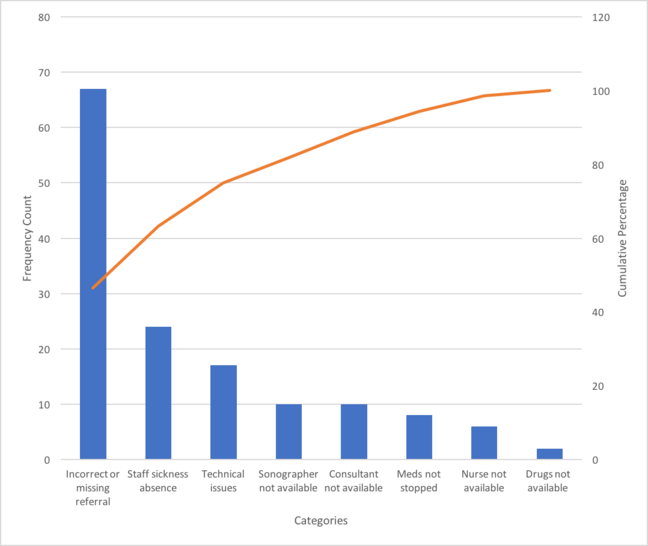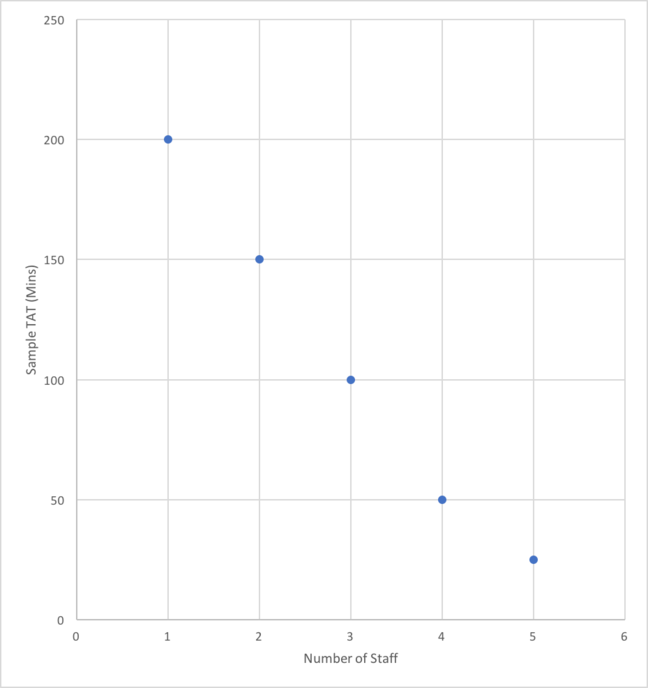3.2

## Manchester Metropolitan University# Measuring the current service to identify the problems

In this step, we will discuss techniques used to measure current service and formulate a baseline. In this context a baseline is what happens now, for example the current waiting list length or the time taken to analyse samples in your laboratory. Knowing this baseline is necessary to allow you to measure and quantify the impact of the change you have introduced, for example it has resulted in shorter waiting list time or time taken to analyse samples in your laboratory.

## The histogram

The histogram, is a graphical representation of data in the form of bars and is sometimes referred to as a bar chart. The histogram is an excellent way to graphically represent numerical data such as turnaround time for test results.

For example, the histogram in the example below shows fictional data for sample turnaround time (mins) in a blood science laboratory. As you can see, the sample turnaround time varies throughout the day, related to the volume of work arriving in the department, from outpatients and family doctors. While the data here is fictional it illustrates how a simple graphical representation can make it easy to spot patterns.

Histograms can be created very easily in any spreadsheet programme.© Garry McDowell

## Pareto Chart

This is a variation on the histogram and is based on what is known as the Pareto principle, or the 80/20 rule. Basically, what the 80/20 rule means is that in any group of factors that contribute to an effect, 80% of the effect comes from 20% of the causes.

Developing a Pareto Chart

Stage 1: Collect data

• Compile a simple table, listing all the contributing factors to a particular effect and the magnitude of the effect.
• Order the contributing factors according to frequency, starting with the highest and working down.
• Calculate percentage of the total for each.
• Working from highest to lowest, calculate the cumulative percentage for each until you reach 100%

Stage 2: Draw Pareto Chart

• The left vertical (y) axis should be the frequency of the effect (for example number of errors, number of cancellations by way of example)
• The horizontal (x) axis is the categories
• The right vertical (y) axis is the cumulative percentage.
• Draw a bar chart (histogram) to show the frequency
• Draw a line graph, on the same graph, to show the cumulative percentage.
• Lets look at an example. Below is some fictional data related to cancelled echocardiography sessions table in the Related Files shows the categories of causes, percentage and cumulative percentage. The associated Pareto chart is shown below:© Garry McDowell

Again, you can draw these diagrams easily by hand, but use the technology to help you. The chart above was generated using a standard readily available spreadsheet.

## Scatter Diagram

A scatter diagram is a graphical representation of the relationship between two continuous variables. They can be used to look at the cause and effect between two variables. Let us look at an example. Suppose your brainstorming sessions highlighted staff shortage as a cause of increased turnaround time and you wished to evaluate the relationship between the number of staff on duty and the sample turnaround time in a blood science department. The scatter diagram is a simple representation of both these variable. An example is shown below.© Garry McDowell

## Discussion

Will any of the tools described above help you in establishing the baseline for your proposed innovation? Use the comments section to write a few words about the tool you think is most appropriate. Reflect on other comments and consider if their choices are appropriate.